# mathr / blog / #

## Atom domain size estimation

The Mandelbrot set is defined by iterations of a quadratic polynomial:

\begin{aligned} F(z,c) &= z^2 + c \\ F^{n+1}(z, c) &= F^{n}(F(z,c),c) \end{aligned}

Atom domain representation for the Mandelbrot set colours points according to "near-miss" periods. The definition I'll use for $$a(c)$$ atom period of a point is:

$a(c) = q \text{ where } 1 \le q \le p \text{ minimizes } |F^q(0,c)|$

taking $$p$$ to be the period of the hyperbolic component containing $$c$$ for interior points, or $$\infty$$ for exterior points.

The atom periods of nearby points are likely to be the same, and the restricted atom periods (limiting the maximum iteration count to the desired atom period) form connected atom domains. These domains may be expressed as:

$\{ c : |F^p(0,c)| \lt \min_{q=1}^{p-1} \{ |F^q(0,c) \} \}$

The interior of each restricted atom domain with period $$p$$ contains a nucleus $$c_0$$ of period $$p$$ with $$F^p(0,c_0) = 0$$. On the boundary of the domain, $$|F^p(0,c)| = |F^q(0,c)|$$ for some $$1 \le q \lt p$$, which implies $$1 = |F^p(0,c)/F^q(0,c)| =: |G(c)|$$. Assuming $$q$$ to be constant throughout the whole domain, and approximating $$G$$ by a power series at $$c = c_0 + h$$ near the nucleus $$c_0$$, gives:

$G(c) = G(c_0) + h \frac{\partial G}{\partial c}(c_0) + O(h^2)$

Using the quotient rule $$(f/g)' = (f' g - f g')/g^2$$ and observiing that $$G(c_0) = 0$$ results in

$\frac{\partial G}{\partial c}(c) = \frac{ \frac{\partial F^p}{\partial c} (0,c) }{ F^q(0, c) }$

Taking $$|G(c)| = 1$$ and assuming $$h$$ is small enough to ignore the $$O(h^2)$$ tail of the series gives an estimate for the size $$R_a$$ of the atom domain:

$R_a = |h| = \left|\frac{ F^q(0,c_0) }{ \frac{\partial F^p}{\partial c}(0,c0) }\right|$

So, given a nucleus $$c$$ and its period $$p$$ the size estimate of its atom domain can easily be calculated:

real_t atom_domain_size_estimate(complex_t c, int_t p) {
complex_t z = c;
complex_t dc = 1;
real_t abszq = cabs(z);
for (int_t q = 2; q <= p; ++q) {
dc = 2 * z * dc + 1;
z = z * z + c;
real_t abszp = cabs(z);
if (abszp < abszq && q < p) {
abszq = abszp;
}
}
return abszq / cabs(dc);
}

But, how good is this approximate estimate? I checked it graphically. In these images, I used interior and exterior distance estimation (wiggly grey boundary of the mandelbrot set), and edge detection filter on the (unrestricted) atom domains (smooth grey arcs in the exterior of the set). I calculated the nucleus and period for each of the larger domains, and drew a circle around the nucleus (labeled with its period) with radius of the size estimate. A rectangle shows the location of the next image.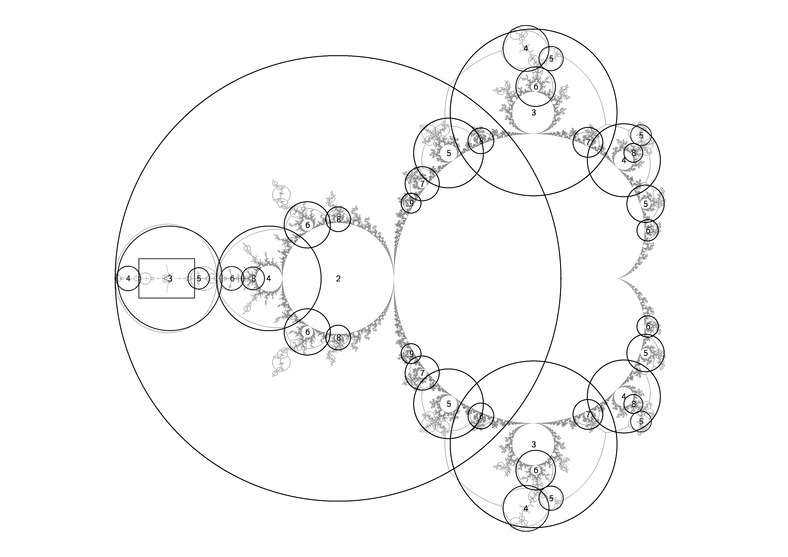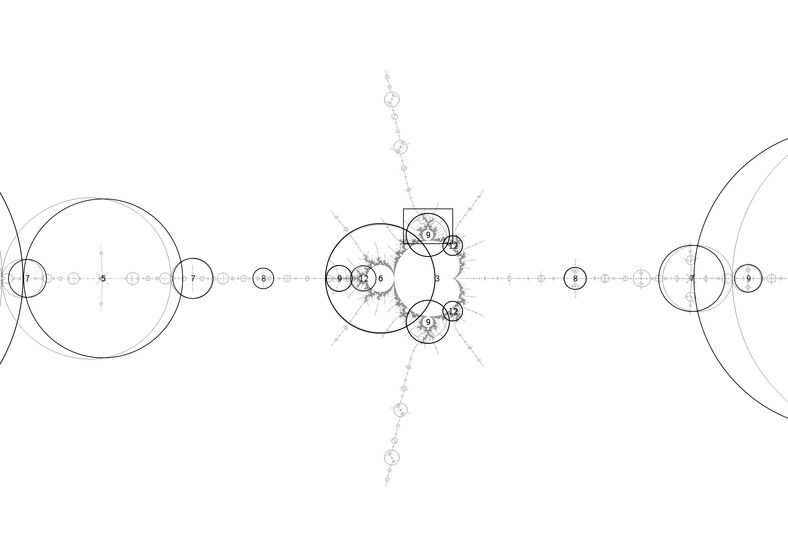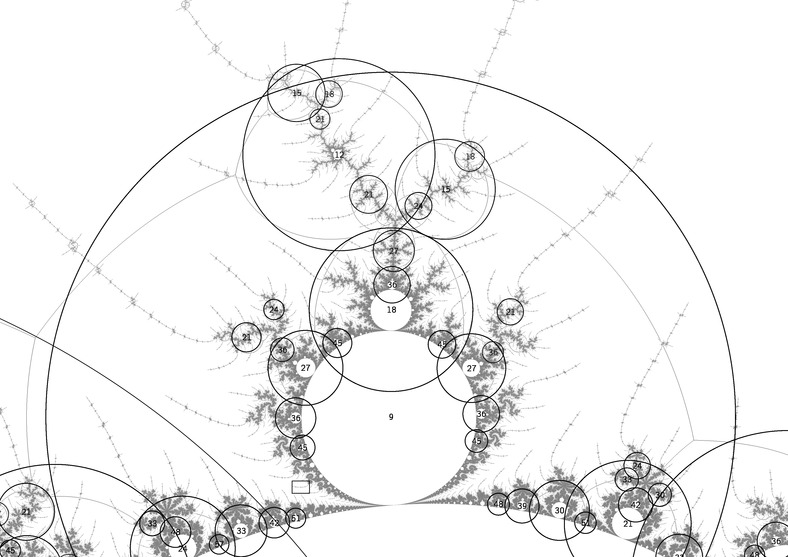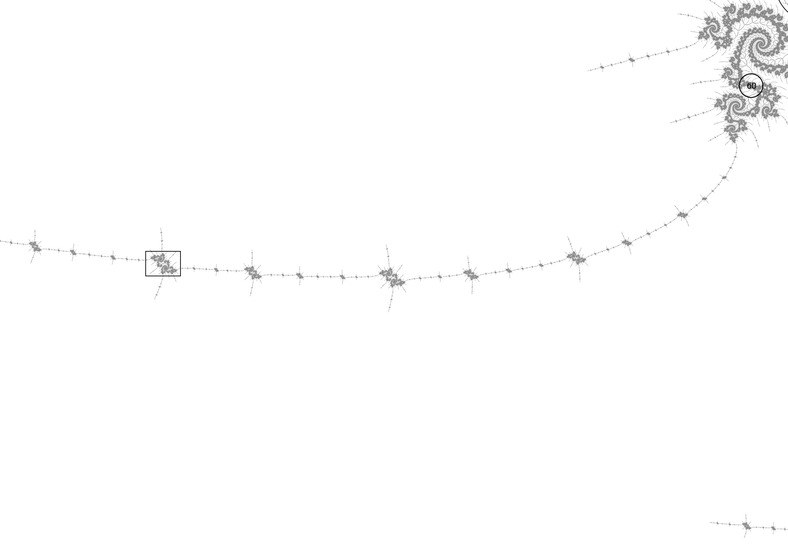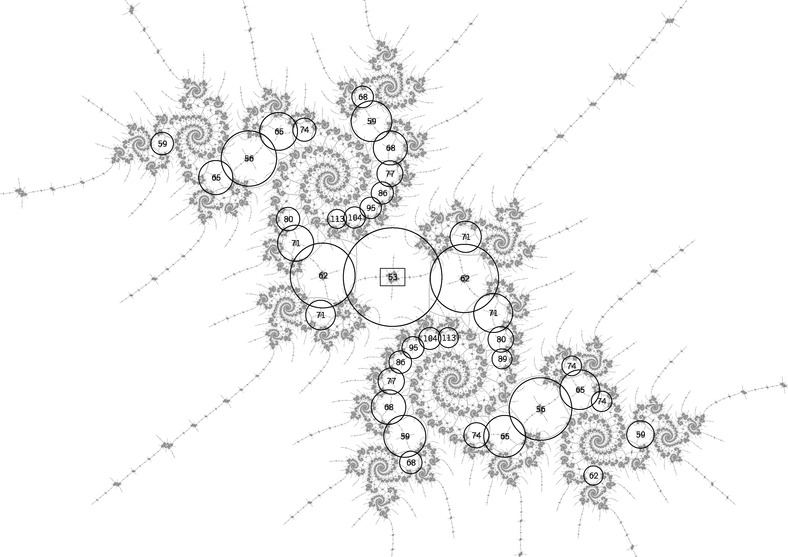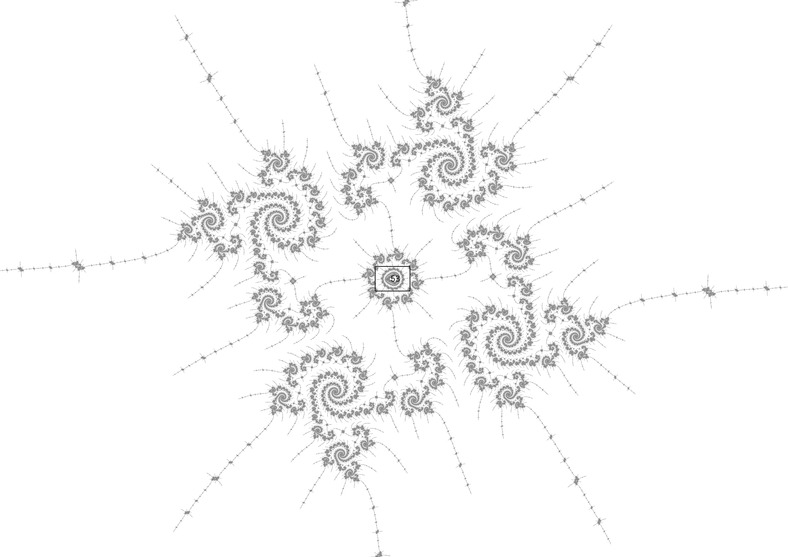They seem to match up pretty well!